# PSAT Math: Triangles & Trigonometric Ratios Chapter Exam

Exam Instructions:

Choose your answers to the questions and click 'Next' to see the next set of questions. You can skip questions if you would like and come back to them later with the yellow "Go To First Skipped Question" button. When you have completed the practice exam, a green submit button will appear. Click it to see your results. Good luck!

Answered 0 of 30

### Page 1

#### Question 1 1. Conclude whether triangle BEN is similar to triangle MAT.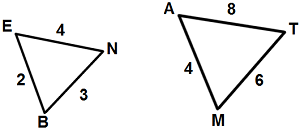#### Question 2 2. The isosceles triangle pictured below shows one angle measuring 40 degrees. What is the measure of angle x?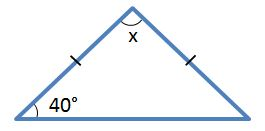#### Question 3 3. Find the value of x, rounded to the nearest degree.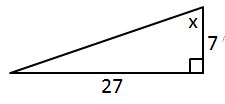#### Question 4 4. What is x?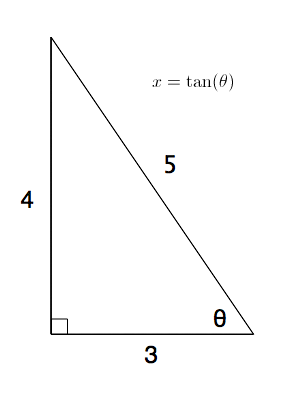#### Question 5 5. Given triangles DAR and KMR. What is the value of y?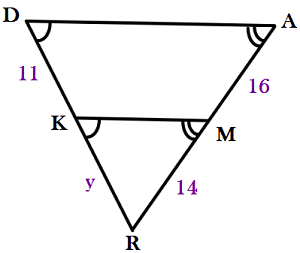### Page 2

#### Question 6 6. What is x?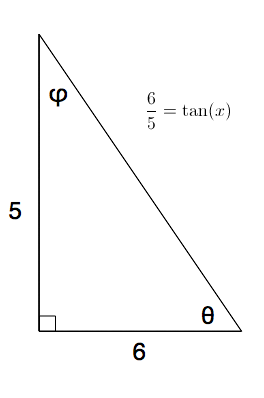#### Question 7 7. What type of triangle is pictured below?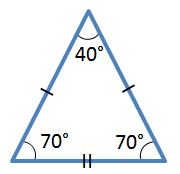#### Question 9 9. In the right triangle pictured below, what is the value of angle x?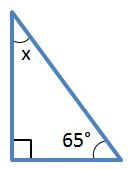#### Question 10 10. Find the value of x, rounded to the nearest tenth.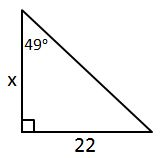### Page 3

#### Question 14 14. What type of triangle is pictured?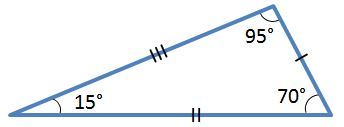#### Question 15 15. The lines a and b are parallel lines of length 5 and 2. The lines d and e are parallel to one another, and both are perpendicular to a and b. What is the length of c?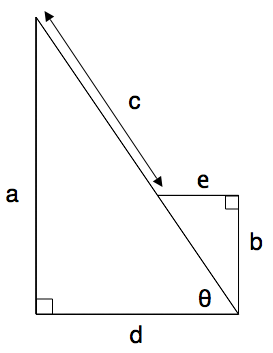### Page 4

#### Question 16 16. In the picture shown below, there are three lines marking each side of the triangle. These lines give the indication that the triangle is: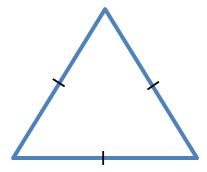#### Question 19 19. Find the value of x, rounded to the nearest tenth.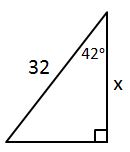#### Question 20 20. What is x?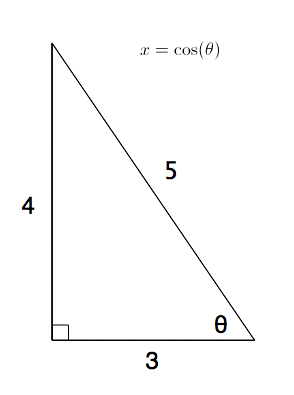### Page 5

#### Question 21 21. Determine if triangle VEZ is similar to triangle MAG.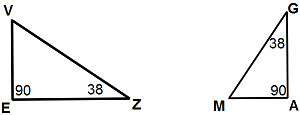#### Question 22 22. Triangle HYV and triangle AYB are similar by the AA similarity theorem. What is the value of x?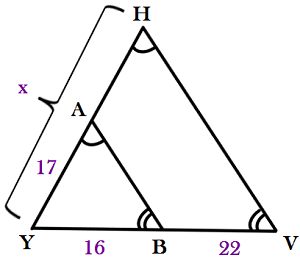#### Question 23 23. In the pictured triangle, what ratio represents the cosine of theta?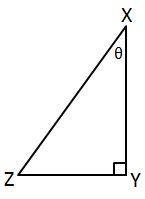#### Question 25 25. Find the value of x, rounded to the nearest degree.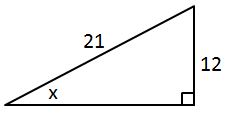### Page 6

#### Question 28 28. What is x?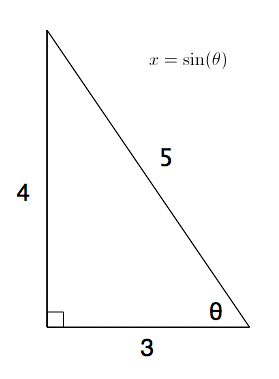#### Question 29 29. Find the value of x, rounded to the nearest tenth.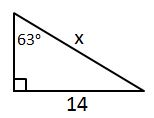#### PSAT Math: Triangles & Trigonometric Ratios Chapter Exam Instructions

Choose your answers to the questions and click 'Next' to see the next set of questions. You can skip questions if you would like and come back to them later with the yellow "Go To First Skipped Question" button. When you have completed the practice exam, a green submit button will appear. Click it to see your results. Good luck!

Support